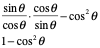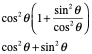# Problem of the Day March 27, 2020

Mar 27, 2020March 27, 2020 Problem of the Day

Grade 6: Solve the following problems: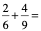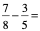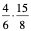=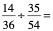Grace 7: Solve the following equations:

2x - 3 = 15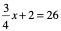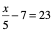4x + 9 = 57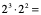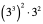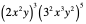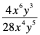Algebra: Solve the following radical equations: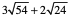=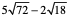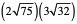Geometry: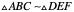solve for the variablesAlgebra 2: Solve the following equations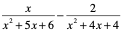Trigonometry:  `Solve the following equations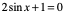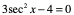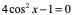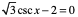Pre-Calculus: Verify the following trigonometric identities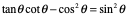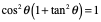Grade 6: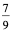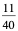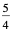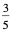Grade 7:   9,   32,   150,   17

Grade 8: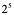,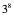,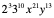,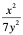Algbebra: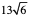,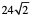,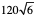Geometry:  x = 8   y = 12   z = 25   m = 42   n = 113

Algebra 2: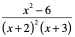Trigonometry: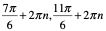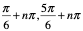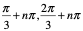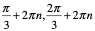Precalculus: